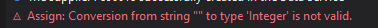# String to Double Datatable LinQ

Hello, I would like to convert rows from String to Double using LinQ query. However I got this error message:Some of my cells are empty… I would like to include a condition: If the cell is empty, then does nothing, otherwise converts. Do you have any solutions for me? Thanks

“CSVDataTable.AsEnumerable.Select(
).CopyToDataTable”

@DimitriLYR

You can change cdbl(r(22)) like this

If(IsNumeric(r(22).ToString),Cdbl(r(22)),0)

Repeat same for all columns

Cheers

1 Like

Thanks for your answer, the function passes, but my values ​​in my file are still stored as text.

@DimitriLYR

Whicha ctivity are you trying?

I beleive column type is already double…

And is the excel column type changed to number as well? If not you can use format range activity

Cheers

Kindly try the below expression-

CorrectedInputDT = (From r In InputDT.AsEnumerable()
Let ab = r.ItemArray.take(18).Concat(r.ItemArray.skip(18).Take(5).ToList().ConvertAll(Function(a) CDbl(“0”+a.ToString.))).ToArray
).CopyToDataTable

Regards

Kindly try the below expression-

``````CorrectedInputDT = (From r In InputDT.AsEnumerable()
Let ab = r.ItemArray.take(18).Concat(r.ItemArray.skip(18).Take(5).ToList().ConvertAll(Function(a) CDbl("0"+a.ToString.))).ToArray
).CopyToDataTable
``````

Regards

1 Like

I’m using the “Read Workbook” workbook read range. My column is in String

1 Like

Hi @DimitriLYR ,

Maybe an Alternate would be to use `Format Cells` Activity :

However, You would require to enable Modern Design and use the Excel Scope Activities to achieve this.

1 Like

This topic was automatically closed 3 days after the last reply. New replies are no longer allowed.